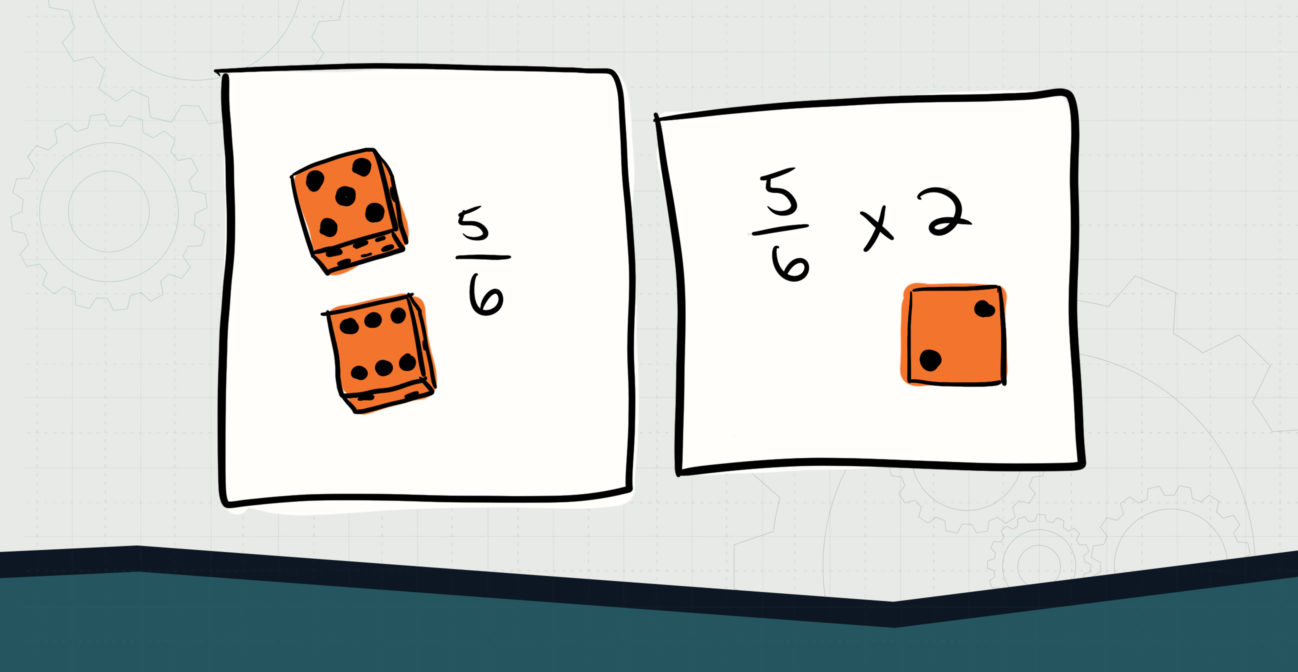# Greater Than One

## Complete fraction multiplication with the assistance of bricks.Objective:
Child will be able to use bricks for beginning fraction multiplication.

Essential Question(s):
When multiplying whole numbers by a fraction, how can we visualize the mathematics?

Special Materials:
Dice, pencil and paper

Bricks Required:

1×2 bricks in three colors, 16×16 plates

Project Structure

Engage/Explain:

Explore:

1. Give child a handful of bricks, a 16×16 plate, and a pair of dice.
2. Child rolls both dice to make a fraction multiplier, smaller number on top, then notes it on their paper.
3. Child rolls one die to determine the whole number multiplicand.
4. Child counts out 1×2 bricks to create their fraction: x number of color A for the numerator and then enough of color B to make the total for the denominator.
5. Child continues counting out fractions until they have as many as the multiplicand, then counts how many of color A they have total.
6. Child then groups as many of color C as color A, according to the denominator, to find the result of their multiplication sentence; they should reduce if necessary.

Elaborate:

1. Ask child to create an explanation on how they are multiplying and visualizing the fractions so they can teach the process to others.
2. Child should also explain the effects of multiplying by a number greater than 1 vs. less than.